Courses

# Test: Theoretical Distributions- 2

## 40 Questions MCQ Test Business Mathematics and Logical Reasoning & Statistics | Test: Theoretical Distributions- 2

Description
This mock test of Test: Theoretical Distributions- 2 for CA Foundation helps you for every CA Foundation entrance exam. This contains 40 Multiple Choice Questions for CA Foundation Test: Theoretical Distributions- 2 (mcq) to study with solutions a complete question bank. The solved questions answers in this Test: Theoretical Distributions- 2 quiz give you a good mix of easy questions and tough questions. CA Foundation students definitely take this Test: Theoretical Distributions- 2 exercise for a better result in the exam. You can find other Test: Theoretical Distributions- 2 extra questions, long questions & short questions for CA Foundation on EduRev as well by searching above.
QUESTION: 1

Solution:
QUESTION: 2

Solution:
QUESTION: 3

### If X and Y are two independent random variables such that X ~ χ2m and Y~ χ2 n , then the distribution of (X +Y) is

Solution:
QUESTION: 4

(Direction 4 - 27) Write down the correct answers. Each question carries 2 marks.

Q. What is the standard deviation of the number of recoveries among 48 patients when the probability of recovering is 0.75?

Solution:
QUESTION: 5

X is a binomial variable with n = 20. What is the mean of X if it is known that x is  symmetric?

Solution:
QUESTION: 6

If X ~ B (n, p), what would be the least value of the variance of x when n = 16?

Solution:
QUESTION: 7

If x is a binomial variate with parameter 15 and 1/3, what is the value of mode of the distribution

Solution:
QUESTION: 8

What is the no. of trials of a binomial distribution having mean and SD as 3 and 1.5 respectively?

Solution:
QUESTION: 9

What is the probability of getting 3 heads if 6 unbiased coins are tossed simultaneously?

Solution:
QUESTION: 10

If the overall percentage of success in an exam is 60, what is the probability that out of a group of 4 students, at least one has passed?

Solution:
QUESTION: 11

What is the probability of making 3 correct guesses in 5 True – False answer type questions?

Solution:
QUESTION: 12

If the standard deviation of a Poisson variate X is 2, what is P (1.5 < X < 2.9)?

Solution:
QUESTION: 13

If the mean of a Poisson variable X is 1, what is P (X = at least one)?

Solution:
QUESTION: 14

If X ~ P (m) and its coefficient of variation is 50, what is the probability that X would assume only non-zero values?

Solution:
QUESTION: 15

If 1.5 percent of items produced by a manufacturing units are known to be defective, what is the probability that a sample of 200 items would contain no defective item?

Solution:
QUESTION: 16

For a Poisson variate X, P (X = 1) = P (X = 2). What is the mean of X?

Solution:
QUESTION: 17

If 1 percent of an airline‘s flights suffer a minor equipment failure in an aircraft, what is the probability that there will be exactly two such failures in the next 100 such flights?

Solution:
QUESTION: 18

If for a Poisson variable X, f(2) = 3 f(4), what is the variance of X?

Solution:
QUESTION: 19

What is the coefficient of variation of x, characterised by the following probability density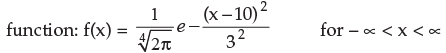Solution:
QUESTION: 20

What is the first quartile of X having the following probability density function?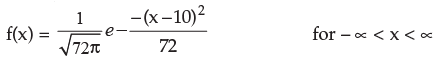Solution:
QUESTION: 21

If the two quartiles of N (µ , σ2) are 14.6 and 25.4 respectively, what is the standard deviation of the distribution?

Solution:
QUESTION: 22

If the mean deviation of a normal variable is 16, what is its quartile deviation?

Solution:
QUESTION: 23

If the points of inflexion of a normal curve are 40 and 60 respectively, then its mean deviation is

Solution:
QUESTION: 24

If the quartile deviation of a normal curve is 4.05, then its mean deviation  is

Solution:
QUESTION: 25

If the Ist quartile and mean deviation about median of a normal distribution are 13.25 and 8 respectively, then the mode of the distribution is

Solution:
QUESTION: 26

If the area of standard normal curve between z = 0 to z = 1 is 0.3413,  then the value of φ (1) is

Solution:
QUESTION: 27

If X and Y are 2 independent normal variables with mean as 10 and 12 and SD as 3 and 4, then (X+Y) is normally distributed with

Solution:
QUESTION: 28

(Direction 28 - 40) Answer the following questions. Each question carries 5 marks.

Q. If it is known that the probability of a missile hitting a target is 1/8, what is the probability that out of 10 missiles fired, at least 2 will hit the target?

Solution:
QUESTION: 29

X is a binomial variable such that 2 P(X = 2) = P(X = 3) and mean of X is known to be 10/3. What would be the probability that X assumes at most the value 2?

Solution:
QUESTION: 30

Assuming that one-third of the population are tea drinkers and each of 1000 enumerators takes a sample of 8 individuals to find out whether they are tea drinkers or not, how many enumerators are expected to report that five or more people are tea drinkers?

Solution:
QUESTION: 31

If a random variable X follows binomial distribution with mean as 5 and satisfying the condition 10 P (X = 0) = P (X = 1), what is the value of P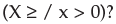Solution:
QUESTION: 32

Out of 128 families with 4 children each, how many are expected to have at least one boy and one girl?

Solution:

Step-by-step explanation:
4children in a family can be in
2 x 2 x 2 x2 = 16 ways
at least one boy and one girl  =
Total cases - all boys - all girs
All boys = 1 case
All girls = 1 case
=> at least one boy and one girl  = 16 - 1 - 1 = 14
Probability of at least one boy and one girl   = 14/16
out of 128 families expected to have = 128  x 14/16  =  8 x 14
= 112
112 Families expected to have at least one boy and one girl.

QUESTION: 33

In 10 independent rollings of a biased die, the probability that an even number will appear 5 times is twice the probability that an even number will appear 4 times. What is the probability that an even number will appear twice when the die is rolled 8 times?

Solution:
QUESTION: 34

If a binomial distribution is fitted to the following data: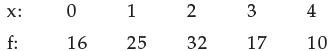then the sum of the expected frequencies for x = 2, 3 and 4 would be

Solution:
QUESTION: 35

If X follows normal distribution with µ = 50 and σ = 10, what is the value of P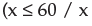> 50)?

Solution:
QUESTION: 36

X is a Poisson variate satisfying the following condition 9 P (X = 4) + 90 P (X = 6) = P (X = 2). What is the value of P (X £ 1)?

Solution:
QUESTION: 37

A random variable x follows Poisson distribution and its coefficient of variation is 50.What is the value of P (x > 1 / x > 0)?

Solution:
QUESTION: 38

A renowned hospital usually admits 200 patients every day. One per cent patients, on an average, require special room facilities. On one particular morning, it was found that only one special room is available. What is the probability that more than 3 patients would require special room facilities?

Solution:
QUESTION: 39

A car hire firm has 2 cars which is hired out everyday. The number of demands per day for a car follows Poisson distribution with mean 1.20. What is the proportion of days on which some demand is refused? (Given e 1.20 = 3.32).

Solution:
QUESTION: 40

If a Poisson distribution is fitted to the following data: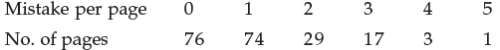Q. Then the sum of the expected frequencies for x = 0, 1 and 2 is

Solution: